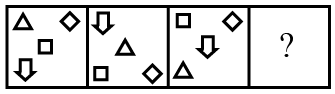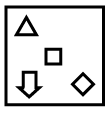Question 8

# Select the figure that can replace the question mark (?) in the following series.Solution

In the given series, the rhombus is moving up and down on the right side. The triangle, square, arrow are rotating in clockwise direction.

$$\therefore\$$The next figure in the series isHence, the correct answer is Option D